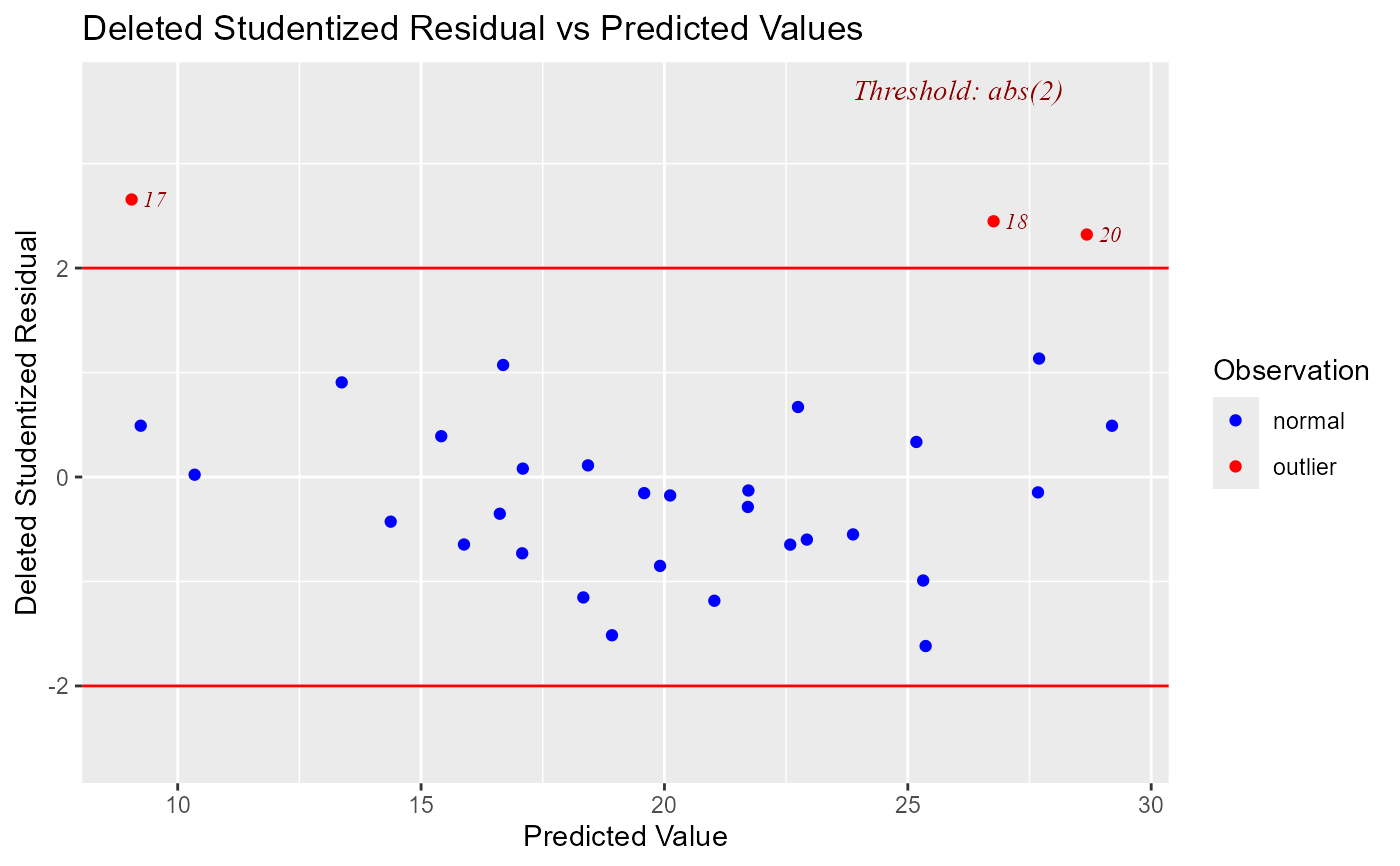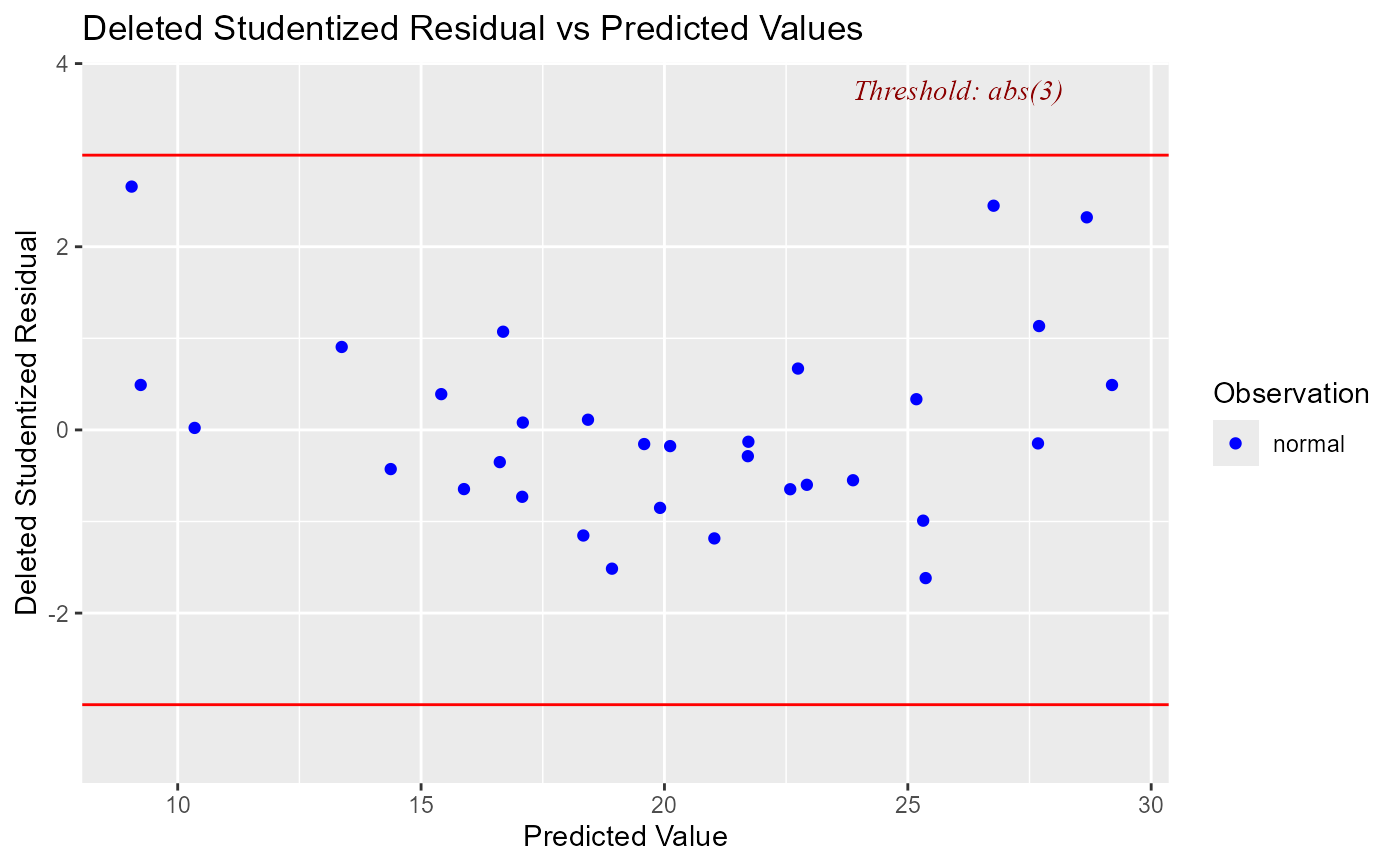Plot for detecting violation of assumptions about residuals such as non-linearity, constant variances and outliers. It can also be used to examine model fit.

## Usage

ols_plot_resid_stud_fit(model, threshold = NULL, print_plot = TRUE)

## Arguments

model

An object of class lm.

threshold

Threshold for detecting outliers. Default is 2.

print_plot

logical; if TRUE, prints the plot else returns a plot object.

## Value

ols_plot_resid_stud_fit returns a list containing the following components:

outliers

a data.frame with observation number, fitted values and deleted studentized residuals that exceed the threshold for classifying observations as outliers/influential observations

threshold

threshold for classifying an observation as an outlier/influential observation

## Details

Studentized deleted residuals (or externally studentized residuals) is the deleted residual divided by its estimated standard deviation. Studentized residuals are going to be more effective for detecting outlying Y observations than standardized residuals. If an observation has an externally studentized residual that is larger than 2 (in absolute value) we can call it an outlier.

model <- lm(mpg ~ disp + hp + wt + qsec, data = mtcars)